Basic Mathematics

# Angles and Lines: Level 2 Challenges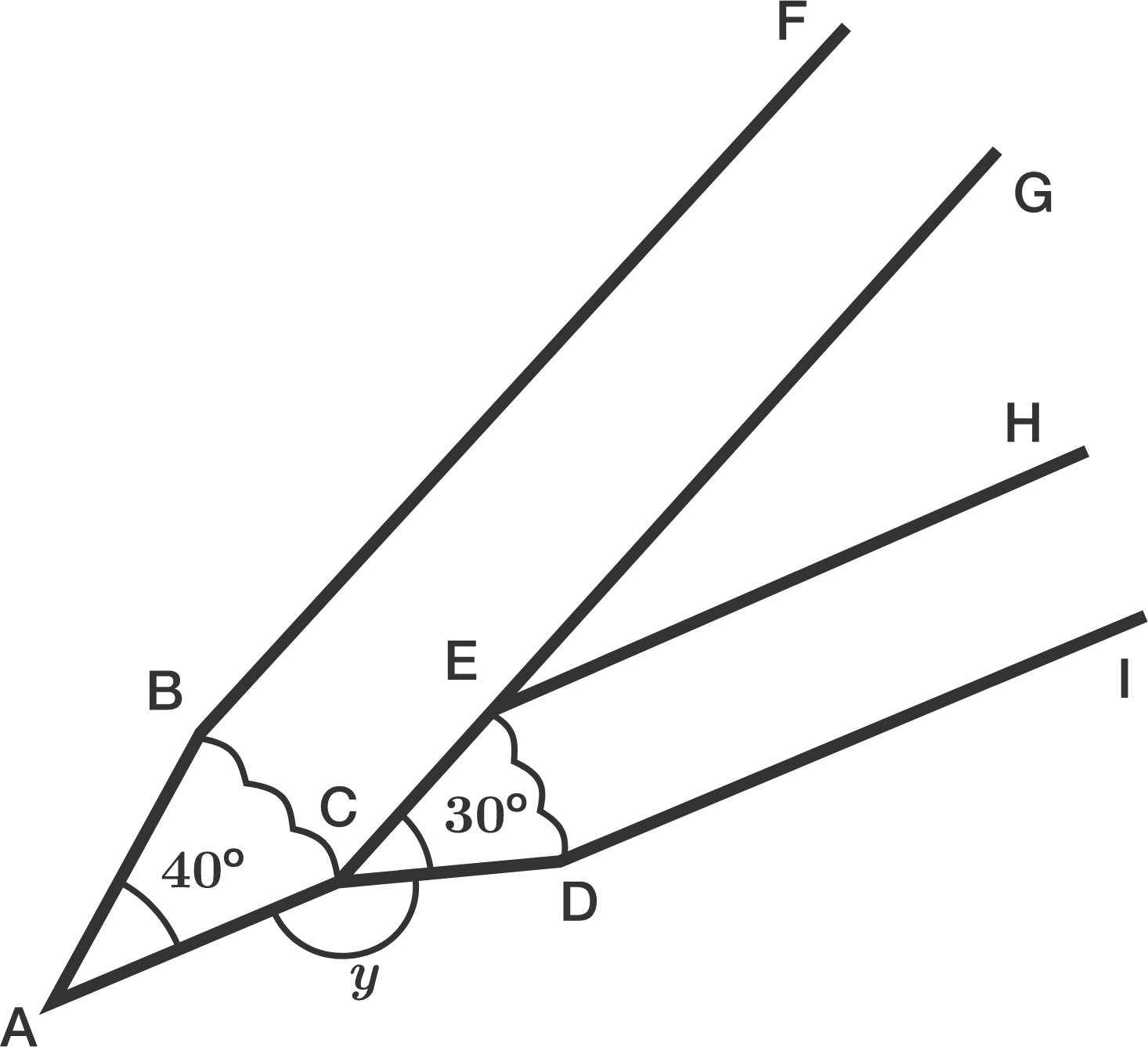Hazri prepared another two pencils and he aligned them as shown in the diagram above.

The two pencils form an angle which is labelled $y$ ($\angle ACD$) in the diagram. If the angle of the pencil tip $A$ is $40^\circ$ and the angle of the pencil tip $ECD$ is $30^\circ$, then what is the value of $y$?

(Assume the pencils have a rectangular body and have their tips resembling isosceles triangles)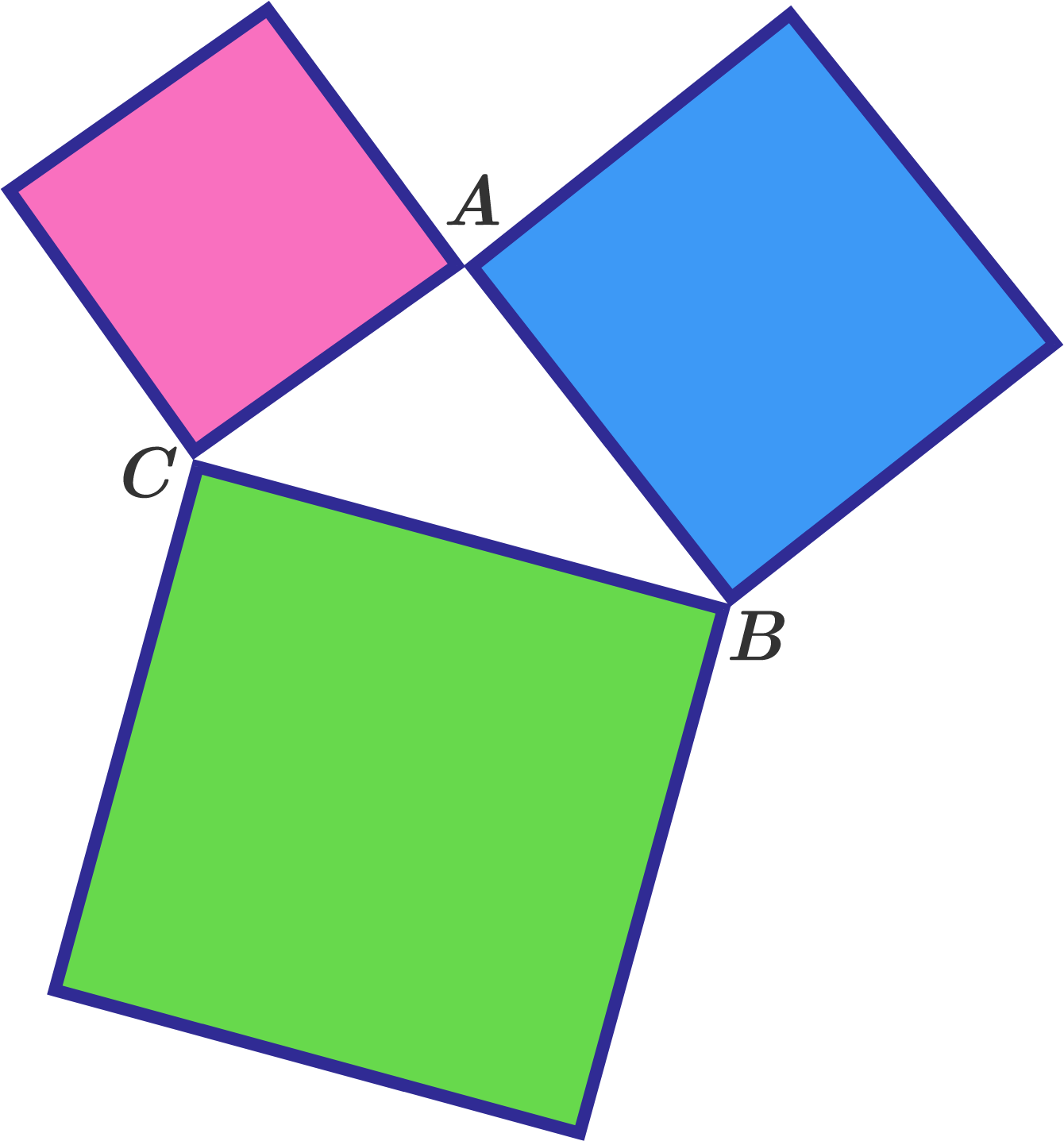Three squares are joined together as shown in figure to create a triangle.

What is the sum of angles $\angle A + \angle B + \angle C$ ?

Note: We are referring to the "exterior angles", not the interior angles of the triangle.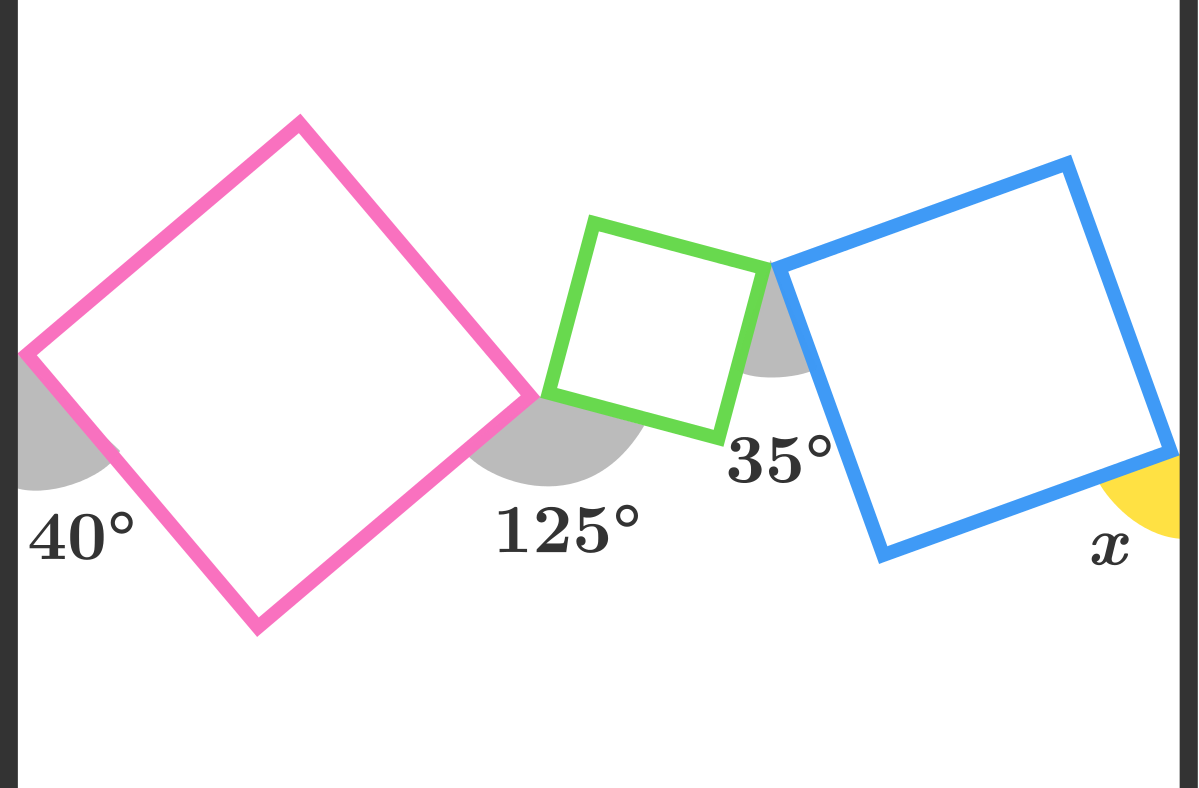As shown in the diagram above, there lie 3 squares between 2 parallel lines such that each pair--(line, square) or (square, square)--just meet at a vertex. Find the measure of angle $x$ in degrees.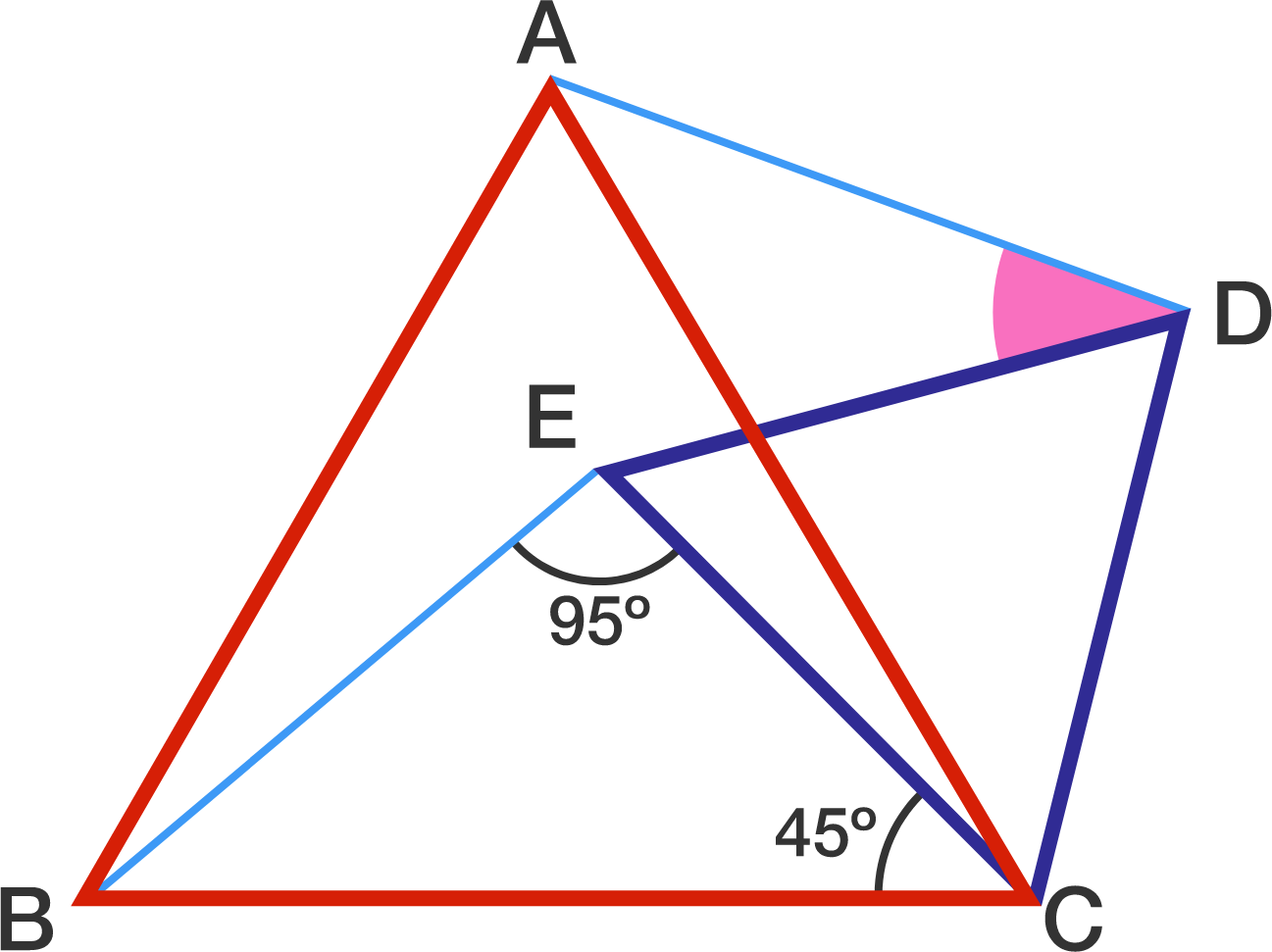In the figure above, $ABC$ and $CDE$ are equilateral triangles. Find $\angle ADE$ in degrees.



###### Source: HKCEE 1991 #51.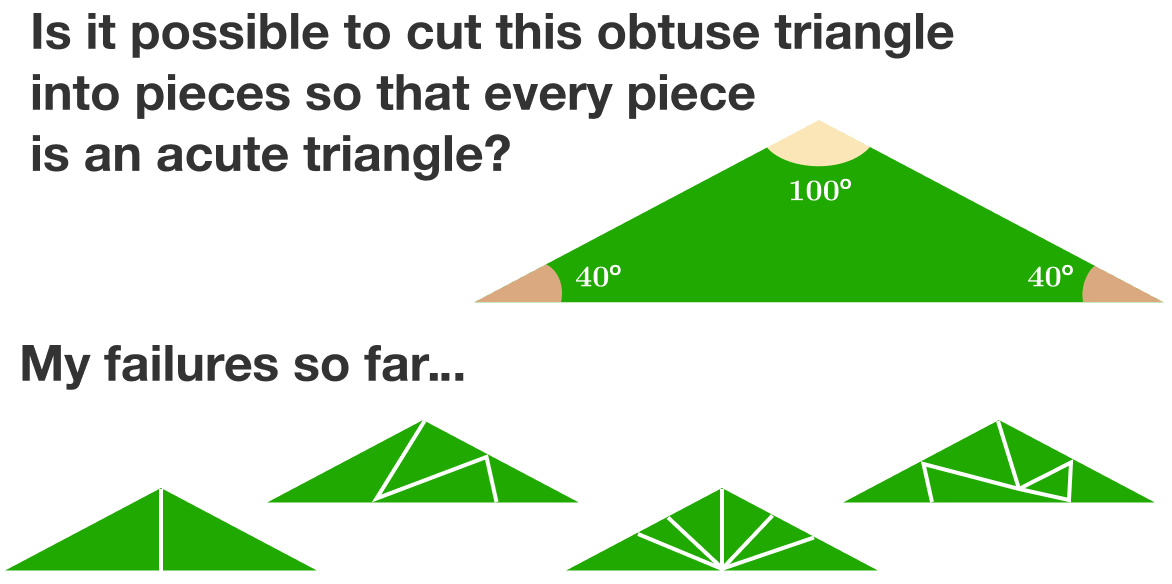×